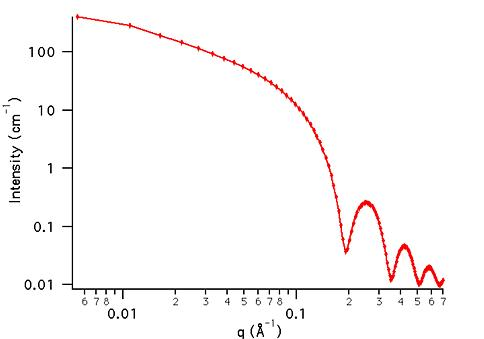MODEL

Cylinder

ORIGINAL AUTHOR/MODIFICATION

Steve Kline 06 NOV 1998

Alan Munter 08 JULY 1999, converted to Java

APPROVED FOR DISTRIBUTION

DESCRIPTION

Calculates the form factor for a monodisperse right circular cylinder with uniform scattering length density. The form factor is normalized by the particle volume such that P(q) = scale*<f2>/Vol + bkg, where < > is an average over all possible orientations of the cylinder.

Resolution smeared version is also provided.

VARIABLES

Input Variables (default values):

Parameter Variable Value
0Scale1.0
2Length (Å)400.0
3Contrast (Å-2)3.0e-6
4Incoherent Background (cm-1)0.0

USAGE NOTES

The function calculated is: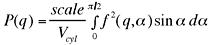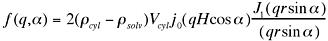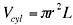and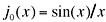where J1(x) is the first order Bessel function. Alpha is defined as the angle between the cylinder axis and the scattering vector, q. The integral over alpha averages the form factor over all possible orientations of the cylinder with respect to q. The returned value is in units of [cm-1], on absolute scale.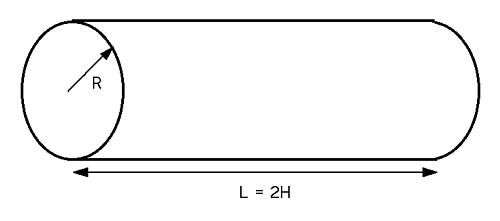Scattering contrast (Dr) = SLD (cylinder) - SLD (solvent)

Parameter (scale) and Parameter (contrast) are both multiplicative factors in the model and are perfectly correlated. One or both of these parameters must be held fixed during model fitting.

If the scale factor Parameter is set equal to the particle volume fraction, phi, the returned value is the scattered intensity per unit volume, I(q) = phi*P(q). However, no interparticle interference effects are included in this calculation.

76-point Gaussian quadrature is used to calculate the form factor. This calcualtion will be slow (approximately 10 seconds) on older machines. The smearing calculation is an additional numerical integration (20-point quadrature) and will further slow the update of the graph.

REFERENCE

Guinier, A. and G. Fournet, "Small-Angle Scattering of X-Rays", John Wiley and Sons, New York, (1955).

TEST DATASET

This example dataset is produced by calculating the Cylinder using 128 data points, qmin = 0.001 Å-1, qmax = 0.7 Å-1 and the above default parameter values.